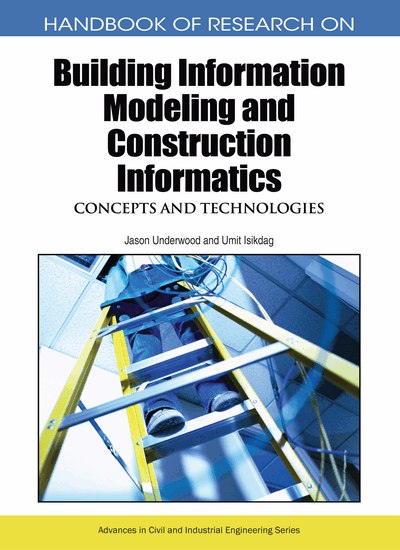# Basic Topological Notions and their Relation to BIM

Norbert Paul (Technische Universität München, Germany)
DOI: 10.4018/978-1-60566-928-1.ch019
Available
\$37.50
No Current Special Offers

## Abstract

Each building sets up a topological space in the mathematical sense. Therefore every Building Information Model (BIM) has to store topological information. Such information can be found, for example, in the IFC (Liebich et al. 2005). The volume modelling part of the IFC uses a so-called ‘IfcTopologyResource’ which is a topological model on the local scope of each single building element. At a global scope, the ‘IfcRelConnects’ class and its subclasses are used for the connectivity of the building parts. This chapter presents a generalizing concept which handles both “local” and “global” connectivity information in a common way and provides means to mutually relate them.
Chapter Preview
Top

## 2 Background

Before we define our topological data model we want to introduce some basic concepts of topology. A large amount of the matter presented in this section can be found in topology textbooks such as, Brown (1988).

### A Naive Approach to Topology

Topology deals with spatial properties, for example, of being “completely within” a set of elements or points (a shape) or at least “close to” it. A space boundary of a room within a building, for instance, is “close to” that room but neither element of the room's space boundary is “within” that room. A door, however, is “within” the shape made of the door itself together with the two rooms it connects. As every shape which has that door “within” itself intersects both rooms, the door is said to be “close” to either of these rooms. In fact, the elementary notions of topology are merely precise definitions of what can be considered “interior”, “boundary”, and “exterior”. We will now specify these concepts for the case of the three-dimensional Euclidean space into which each building is embedded.

## Key Terms in this Chapter

Specialization Preorder: A relation = within a topological space relating each element to the elements it is close to. For example, a door is close to each of the two rooms it connects, hence door = room1 and door = room2. Also a generalization of “connected_to”-relations.

Topology: A structure for a given set (of points or elements) which specifies interior, frontier and exterior of subsets of that set. Formally, a set of subsets of that given set which satisfies the topology axioms (the set of all possible interiors).

Complex, Topological: A topological space which has an algebraic complex associated in a straightforward manner. Then the boundary of an element is considered to “circumscribe” that element (as a cycle): For example, the surface of a body circumscribes its volume.

Continuous function: (continuous map): A function from the points of one topological space to the points of another space which respects the relation of points being close to shapes of that space. Also a generalization of “part_of”-relations.

Topological Database: An integrated family of topological data types, hence a family of topological data types eventually satisfying some additional consistency constraints.

Complex, Algebraic: A series ··· ? Vi ? Vi-1 ? ··· of vector spaces connected by linear functions di: Vi ? Vi-1 such that each composition di-1.di: Vi ? Vi-2 of two consecutive functions always returns zero. Then each image vector di(vi) is considered a “cycle”, as di-1(di(vi))=0

Complex, Relational: Extension of a topological data type: A topological data type which represents both a topological space and its associated algebraic complex.

Topological Space: A set together with a topology for that set.

Topological Construction: General theory of constructing new topological spaces out of given ones by first operating on the point sets and then finding the topology for the resulting set that fits best.

Topological Database Query: The topological construction derived from a relational algebra query operation and adopted to topological data types.

Topological Data Type: A graph used to store the preordered sets (see: specialization preorder) defined by finite topological spaces. The graph itself need not be a preordered set.

## Complete Chapter List

Search this Book:
Reset